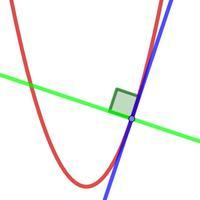# Equation of Tangent and NormalOn this page we look at how to find the equation of a tangent and also the normal to a curve. A tangent is a straight line that touches a curve at one point and has the same gradient as the curve at that point. A normal is straight line...

To access the contents of this site, you need to log in or subscribe